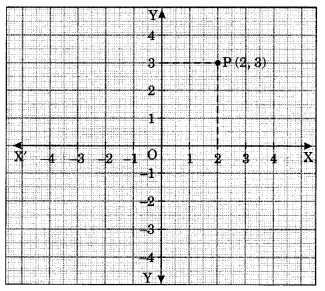On this page, you will find Introduction to Graphs Class 8 Notes Maths Chapter 15 Pdf free download. CBSE NCERT Class 8 Maths Notes Chapter 15 Introduction to Graphs will seemingly help them to revise the important concepts in less time.

## CBSE Class 8 Maths Chapter 15 Notes Introduction to Graphs

### Introduction to Graphs Class 8 Notes Conceptual Facts

• XOX’ and YOY’ taken together are called coordinate axes.
• The plane containing the coordinate axes is called cartesian plane.
• The point of intersection of the coordinate axes is called origin.
• The x-coordinate is always written before y-coordinate e.g., if 2 is x-coordinate and 3 is y-coordinate, then the coordinates are (2, 3).• The x-coordinate of a point lying on y-axis is zero.
• The y-coordinate of a point lying on x-axis is zero.
• The x-coordinate is also called abscissa and y-coordinate ordinate.